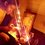# Laws of Binomial theorem (2.1)

Greetings everyone, welcome to the part 2.1 of the Laws of Binomial theorem(2.2 coming next week). In this discussion we will use integration and differentiation to transform our original equation to get a desired series . Check the 1st part before you continue with this one. We are starting with the binomial expansion of $(1+x)^{n}$ . We are calling it equation $I$ . Where $x$ is a complex number and $n$ is a whole number.

$\ (1+x)^{n} = \dbinom{n}{0}x^{0}+\dbinom{n}{1}x^{1}+\dbinom{n}{2}x^{2}+\cdots+\dbinom{n}{n-1}x^{n-1}+\dbinom{n}{n}x^{n} = \displaystyle\sum_{r=0}^{n}\dbinom{n}{r}x^{r}$

Some Important results obtained from $I$ are

$1)$ put $x=1$

$\boxed{ 2^{n} = \dbinom{n}{0}+\dbinom{n}{1}+\dbinom{n}{2}+\cdots+\dbinom{n}{n-1}+\dbinom{n}{n} = \displaystyle\sum_{r=0}^{n}\dbinom{n}{r}2^{r} }$

$2)$ put $x=-1$

$0 = \dbinom{n}{0}-\dbinom{n}{1}+\dbinom{n}{2}-\cdots+\dbinom{n}{n-1}(-1)^{n-1}+\dbinom{n}{n}(-1)^{n} = \displaystyle\sum_{r=0}^{n}\dbinom{n}{r}(-1)^{r}$

$\boxed{ \dbinom{n}{0}+\dbinom{n}{2}+\dbinom{n}{4}+\cdots=\dbinom{n}{1}+\dbinom{n}{3}+\dbinom{n}{5}+\cdots = 2^{n-1} }$

Now , Differentiate $I$ with respect to $x$

$n(1+x)^{n-1} =1\cdot\dbinom{n}{1}+2\cdot\dbinom{n}{2}x^{1}+\cdots+(n-1)\cdot\dbinom{n}{n-1}x^{n-2}+n\cdot\dbinom{n}{n}x^{n-1} = \displaystyle\sum_{r=0}^{n}r\dbinom{n}{r}x^{r-1}$

put $x=1$

$\boxed{ n\cdot2^{n-1} =1\cdot\dbinom{n}{1}+2\cdot\dbinom{n}{2}+\cdots+(n-1)\cdot\dbinom{n}{n-1}+n\cdot\dbinom{n}{n} = \displaystyle\sum_{r=0}^{n}r\dbinom{n}{r} }$

Similarly we can differentiate $I$ twice to get a new series. Let's Try to find the sum of

$1^2\cdot\dbinom{n}{1}+2^2\cdot\dbinom{n}{2}+\cdots+(n-1)^2\cdot\dbinom{n}{n-1}+n^2\cdot\dbinom{n}{n} = \displaystyle\sum_{r=0}^{n}r^2\dbinom{n}{r}$

Let's start with $I$ $\ (1+x)^{n} = \dbinom{n}{0}x^{0}+\dbinom{n}{1}x^{1}+\cdots+\dbinom{n}{n}x^{n} = \displaystyle\sum_{r=0}^{n}\dbinom{n}{r}x^{r} \quad\quad\quad\quad\quad\quad\quad\quad \small\color{#3D99F6}{\text{0th derivative}}$

$n(1+x)^{n-1} =1\cdot\dbinom{n}{1}+2\cdot\dbinom{n}{2}x^{1}+\cdots+n\cdot\dbinom{n}{n}x^{n-1} = \displaystyle\sum_{r=0}^{n}r\dbinom{n}{r}x^{r-1} \quad \small\color{#3D99F6}{\text{1st derivative}}$

$nx(1+x)^{n-1} =1\cdot\dbinom{n}{1}x^{1}+2\cdot\dbinom{n}{2}x^{2}+\cdots+n\cdot\dbinom{n}{n}x^{n} = \displaystyle\sum_{r=0}^{n}r\dbinom{n}{r}x^{r} \quad \small\color{#3D99F6}{\text{times x on both sides}}$

$\underbrace{n(1+x)^{n-1}+n(n-1)x(1+x)^{n-2}}_{n(nx+1)(1+x)^{n-2}} =1^2\cdot\dbinom{n}{1}+2^2\cdot\dbinom{n}{2}x^1+\cdots+n^2\cdot\dbinom{n}{n}x^{n-1} = \displaystyle\sum_{r=0}^{n}r^2\dbinom{n}{r}x^{r-1} \quad \small\color{#3D99F6}{\text{2nd derivative}}$

$\boxed{ n(n+1)2^{n-2}=1^2\cdot\dbinom{n}{1}+2^2\cdot\dbinom{n}{2}+\cdots+n^2\cdot\dbinom{n}{n}= \displaystyle\sum_{r=0}^{n}r^2\dbinom{n}{r} \quad \small\color{#3D99F6}{\text{Put x=1}} }$

For Practice try to find the sum of the following series:

$1) \quad 1^3\cdot\dbinom{n}{1}+2^3\cdot\dbinom{n}{2}+\cdots+(n-1)^3\cdot\dbinom{n}{n-1}+n^3\cdot\dbinom{n}{n}$

$2) \quad 1\cdot2\cdot\dbinom{n}{2}+3\cdot2\cdot\dbinom{n}{3}+\cdots+(n-1)\cdot(n-2)\cdot\dbinom{n}{n-1}+n\cdot(n-1)\dbinom{n}{n}$

Thank You :)Note by Sabhrant Sachan
4 years, 6 months ago

This discussion board is a place to discuss our Daily Challenges and the math and science related to those challenges. Explanations are more than just a solution — they should explain the steps and thinking strategies that you used to obtain the solution. Comments should further the discussion of math and science.

When posting on Brilliant:

• Use the emojis to react to an explanation, whether you're congratulating a job well done , or just really confused .
• Ask specific questions about the challenge or the steps in somebody's explanation. Well-posed questions can add a lot to the discussion, but posting "I don't understand!" doesn't help anyone.
• Try to contribute something new to the discussion, whether it is an extension, generalization or other idea related to the challenge.
• Stay on topic — we're all here to learn more about math and science, not to hear about your favorite get-rich-quick scheme or current world events.

MarkdownAppears as
*italics* or _italics_ italics
**bold** or __bold__ bold
- bulleted- list
• bulleted
• list
1. numbered2. list
1. numbered
2. list
Note: you must add a full line of space before and after lists for them to show up correctly
paragraph 1paragraph 2

paragraph 1

paragraph 2

[example link](https://brilliant.org)example link
> This is a quote
This is a quote
    # I indented these lines
# 4 spaces, and now they show
# up as a code block.

print "hello world"
# I indented these lines
# 4 spaces, and now they show
# up as a code block.

print "hello world"
MathAppears as
Remember to wrap math in $$ ... $$ or $ ... $ to ensure proper formatting.
2 \times 3 $2 \times 3$
2^{34} $2^{34}$
a_{i-1} $a_{i-1}$
\frac{2}{3} $\frac{2}{3}$
\sqrt{2} $\sqrt{2}$
\sum_{i=1}^3 $\sum_{i=1}^3$
\sin \theta $\sin \theta$
\boxed{123} $\boxed{123}$

## Comments

Sort by:

Top Newest

Woah, these identities are really nice and useful! I can see combinatorial proofs being used here.

I think you should create a wiki page to showcase these few identities and how to extrapolate these pattern.

- 4 years, 5 months ago

Log in to reply

@Sambhrant Sachan Agreed! Let me know if you need help getting started. Several of these could be added directly to binomial theorem.

Staff - 4 years, 5 months ago

Log in to reply

As soon as i finish my series , i will work on the wiki :) .

- 4 years, 5 months ago

Log in to reply

Sir, can you confirm that total partitions (with permutations) of a integer $N$ is $2^{N-1}$ .

For example ,

\begin{aligned} 3 & = 1+1+1 \quad \quad 2^{3-1} = \boxed{4}\\ & = 1+2 \\ & = 2+1 \\ & = 3 \\ 4 & = 1+1+1+1 \quad \quad 2^{4-1} = \boxed{8} \\ & = 1+1+2 \\ & = 1+2+1 \\ & = 2+1+1 \\ & = 1+3 \\ & = 3+1 \\ & = 2+2 \\ & = 4 \end{aligned}

If it is correct i will explain the proof in combinatorics .

- 4 years, 5 months ago

Log in to reply

Yes it is. It follows directly from product rule.

I think the product rule wiki or the bijection, injection, and surjection wiki would be a much better place for it.

Staff - 4 years, 5 months ago

Log in to reply

×

Problem Loading...

Note Loading...

Set Loading...